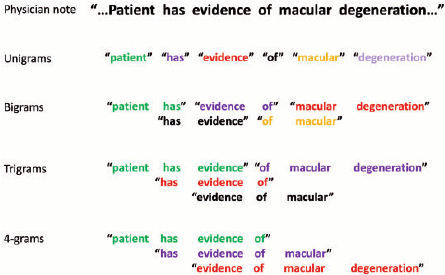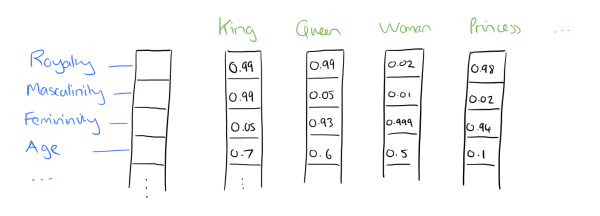(Tim Lee) #1

Unofficial Lecture 10 Notes

Hi all, hope these notes are useful. This is from the morning version of the lecture.

• Tim

Logistic Regression

``````%reload_ext autoreload
%matplotlib inline

from fastai.nlp import *
from sklearn.linear_model import LogisticRegression
# from torchtext import vocab, data, datasets
``````
``````PATH='aclImdb/'
names = ['neg','pos']
``````
``````trn,trn_y = texts_from_folders(f'{PATH}train',names)
val,val_y = texts_from_folders(f'{PATH}test',names)
``````
``````veczr = CountVectorizer(tokenizer=tokenize)
``````
``````trn_term_doc = veczr.fit_transform(trn)
val_term_doc = veczr.transform(val)
``````
``````x=trn_term_doc
y=trn_y
``````

Recapping the Logistic Regression (C = constant)

Make sure you have a lot of parameters. Linear models can work sometimes, if the underlying assumption of linearity isn’t too bad. Works much better with neural networks and more hidden layers.

For sentiment, if you look at a set of words, only need a few words to tell us if the documents is either happy or sad. Have lots of parameters and use regularization

``````m = LogisticRegression(C=1e8, dual=True)
m.fit(x, y)
preds = m.predict(val_term_doc)
(preds==val_y).mean()
``````
``````0.85511999999999999
``````

Trigrams``````veczr =  CountVectorizer(ngram_range=(1,3), tokenizer=tokenize, max_features=800000)
trn_term_doc = veczr.fit_transform(trn)
val_term_doc = veczr.transform(val)
``````
``````x=trn_term_doc
y=trn_y
``````

Note: the `x` input is all 0, or counts

``````
x = [[3 0 0 1...
0 0 0 1
0 2 1 0   ]]

``````
``````m = LogisticRegression(C=1e8, dual=True)
m.fit(x, y)
preds = m.predict(val_term_doc)
(preds==val_y).mean()
``````
``````0.89903999999999995
``````

From Naive Bays

``````y=trn_y
x=trn_term_doc.sign()
val_x = val_term_doc.sign()

p = x[y==1].sum(0)+1
q = x[y==0].sum(0)+1
r = np.log((p/p.sum())/(q/q.sum()))
b = np.log(len(p)/len(q))
``````
``````vocab = veczr.get_feature_names()
``````

How happy or sad everything is

``````np.exp(r)
``````
``````matrix([[ 0.95208,  0.85605,  0.78485, ...,  3.01678,  0.5028 ,  0.5028 ]])
``````
``````vocab[-3]
``````
``````'” .'
``````
``````vocab
``````
``````'! ! "'
``````

All the ones will be replaced with the log count ratio

Now consider a different input

``````
x = [[0 0    0    0.45 ...
0 0    0    0.45
0 0.65 0.45 0   ]]

``````
``````x_nb = x.multiply(r)
m = LogisticRegression(dual=True, C=0.1)
m.fit(x_nb, y);

val_x_nb = val_x.multiply(r)
preds = m.predict(val_x_nb)
(preds.T==val_y).mean()
``````
``````0.91768000000000005
``````

Why is the binarized version better

the r measure is a good estimate of how positive or negative it is. Regularization moves coefficients toward 0. 0 is your prior is your expectation about what hte world is like:

``````Feature Vector
[1 1 0 0
1 0 0 0
1 0 0 1 ]

r = [0 .4 0 .4]
``````
``````Feature Vector x r (positive / negative vectors)
[0 .4 0   0
0  0 0   0
0  0 0 -.4]
``````

Whats the difference between training on (Feature Vector) vs. (Feature Vector x r)

Since the multiplied version. If the cofficients are the same use the R value, which is naive bayes. Otherwise there’s a subtle starting point difference. And under regularization, there’s a varying effect, and will apply variables differently.

Stronger Still

maximum number of unique words in a review

``````sl = 2000
``````
``````md = TextClassifierData.from_bow(trn_term_doc, trn_y, val_term_doc, val_y, sl)
``````
• `wds` - weight decay
• `learning rate : 0.02` - learning rate
``````learner = md.dotprod_nb_learner()
learner.fit(0.02,1,wds=1e-6, cycle_len=1)
``````

Failed to display Jupyter Widget of type `HBox`.

If you're reading this message in the Jupyter Notebook or JupyterLab Notebook, it may mean that the widgets JavaScript is still loading. If this message persists, it likely means that the widgets JavaScript library is either not installed or not enabled. See the Jupyter Widgets Documentation for setup instructions.

If you're reading this message in another frontend (for example, a static rendering on GitHub or NBViewer), it may mean that your frontend doesn't currently support widgets.

``````[ 0.       0.02598  0.11969  0.91618]
``````

2nd iteration

``````learner.fit(0.02,1,wds=1e-6, cycle_len=1)
``````

Failed to display Jupyter Widget of type `HBox`.

If you're reading this message in the Jupyter Notebook or JupyterLab Notebook, it may mean that the widgets JavaScript is still loading. If this message persists, it likely means that the widgets JavaScript library is either not installed or not enabled. See the Jupyter Widgets Documentation for setup instructions.

If you're reading this message in another frontend (for example, a static rendering on GitHub or NBViewer), it may mean that your frontend doesn't currently support widgets.

``````[ 0.       0.02027  0.1131   0.92104]
``````

3Rd iteration

``````learner.fit(0.02,1,wds=1e-6, cycle_len=1)
``````

Failed to display Jupyter Widget of type `HBox`.

If you're reading this message in the Jupyter Notebook or JupyterLab Notebook, it may mean that the widgets JavaScript is still loading. If this message persists, it likely means that the widgets JavaScript library is either not installed or not enabled. See the Jupyter Widgets Documentation for setup instructions.

If you're reading this message in another frontend (for example, a static rendering on GitHub or NBViewer), it may mean that your frontend doesn't currently support widgets.

``````[ 0.       0.01779  0.11128  0.92225]
``````

A Peak under the hood

``````class DotProdNB(nn.Module):
super().__init__()
self.w.weight.data.uniform_(-0.1,0.1)
self.r = nn.Embedding(nf+1, ny)

def forward(self, feat_idx, feat_cnt, sz):
w = self.w(feat_idx)
r = self.r(feat_idx)
return F.softmax(x)

``````

This looks very similar to the work we have done before

The weight adjustment, if not provided, will be `w_adj=0.4`, and for scaling, we will use `r_ad=10`. These constants have been identifyed through experimentation

``````def __init__(self, nf, ny, w_adj=0.4, r_adj=10):

``````

Matrix multiplication

`````` x = ((w+self.w_adj)*r/self.r_adj).sum(1) <-- this is just a matrix multiplication

``````

Let’s talk about whats happening underneath

We want to construct a logistic regression. And currently what we have is a bag of words. But memory becomes an issue now. Even a small corpus can have a large number of documents and a large number of features (unique words).

Every unique word is 1-hot encoded:

``````
terms:
he is a dog
[1  0 0  0
0  1 0  0
0  0 1  0
0  0 0  1 ]

Doc representation

he is a dog ..... more words
docs [                              ]
docs [      big matrix x big matrix ]
docs [                              ]
docs [                              ]
``````

If we multiply the 1-hot encoded by the weight matrix. Selecting that column. A 1-hot matrix multiplier. Looking up a column in an array.

More efficient way:

Don’t store the 1-hot encoded variables. Instead we store the docs in a single vector with the term_index (see below)

``````doc1: a dog is he : [3,4,2,1]
doc2: he is dog : [1,2,4]
doc3: dog is dog : [4,2,4]
``````

1 row for every feature, how every many activations, lets look at each of those word indexes and grab the column out of the weights. This is in contrast to doing the long matrix multiplication

And these docs will pull the corresponding vector. Each weight vector is the

Structured data

Covering Rossman from Deep Learning 3

Turning time series into an embedding? How big / how wide?

Cardinality of the features:

``````[('Store', 1116),
('DayOfWeek', 8),
('Year', 4),
('Month', 13),
('Day', 32),
('StateHoliday', 3),
('CompetitionMonthsOpen', 26),
('Promo2Weeks', 27),
('StoreType', 5),
('Assortment', 4),
('PromoInterval', 4),
('CompetitionOpenSinceYear', 24),
('Promo2SinceYear', 9),
('State', 13),
('Week', 53),
('Events', 22),
('Promo_fw', 7),
('Promo_bw', 7),
('StateHoliday_fw', 4),
('StateHoliday_bw', 4),
('SchoolHoliday_fw', 9),
('SchoolHoliday_bw', 9)]
``````

Embedding sizes (per each field)

``````[(1116, 50),
(8, 4),
(4, 2),
(13, 7),
(32, 16),
(3, 2),
(26, 13),
(27, 14),
(5, 3),
(4, 2),
(4, 2),
(24, 12),
(9, 5),
(13, 7),
(53, 27),
(22, 11),
(7, 4),
(7, 4),
(4, 2),
(4, 2),
(9, 5),
(9, 5)]
``````

But First lets look at the feature engineering

Feature engineering - Rossman

Bring everything you can think of!

``````train, store, store_states, state_names, googletrend, weather, test = tables
``````

Turn the features into human readable

``````train.StateHoliday = train.StateHoliday!='0'
test.StateHoliday = test.StateHoliday!='0'
``````

A small wrapper for the `pandas.DataFrame.merge` or LEFT JOIN

``````def join_df(left, right, left_on, right_on=None, suffix='_y'):
if right_on is None: right_on = left_on
return left.merge(right, how='left', left_on=left_on, right_on=right_on,
suffixes=("", suffix))
``````
• Could have the wrong number of rows
• or missing indexes
``````store = join_df(store, store_states, "Store")
len(store[store.State.isnull()])
``````

Also note, check that don’t lose rows from doing repeated left joins. Also avoid cartesian joins (non-unique join fields) aka MAKE new rows. Also from the merge, be sure to remove the duplicate columns

Memory:

If your joins take too long, consider loading into a SQL database for better joins. Pandas is all in ram, and SQL is designed not to work all in memory, so can handle larger datasets.

Though when working from strings, you may consider doing hte processing in pandas, as you have full access to the python string library tools

Data that was added by joins:

1. state names
3. parse out the different date elements, such as day of the week, month etc.
5. google trend for all of germany
6. days since last competition open since (calculation)

Categorical vs. Continuous

If we treat something as a category, we will be giving it an embedding matrix. We will essentially be developing a description vector. If its continuous, we need to find a underlying function that relates to it. Words easily fall into this categorical.

Some more examples of embeddings. Note that these different color categories are arbitrary and must be interpretted after the fact. The dimension (how many colors, or royalty etc. ) must be chosen beforehand and trained on a deep learningHow do you deal with dates?Turning day of the week data into an embedding

Proximity to holiday. Either upcoming or previous.

• How long has it been since the last promotion?
• How long till the next promotion?
``````def get_elapsed(fld, pre):
day1 = np.timedelta64(1, 'D')
last_date = np.datetime64()
last_store = 0
res = []

for s,v,d in zip(df.Store.values,df[fld].values, df.Date.values):
if s != last_store:
last_date = np.datetime64()
last_store = s
if v: last_date = d
res.append(((d-last_date).astype('timedelta64[D]') / day1).astype(int))
df[pre+fld] = res
``````

Using the function for state holiday and school holiday

``````fld = 'SchoolHoliday'
df = df.sort_values(['Store', 'Date'])
get_elapsed(fld, 'After')
df = df.sort_values(['Store', 'Date'], ascending=[True, False])
get_elapsed(fld, 'Before')

``````
``````fld = 'StateHoliday'
df = df.sort_values(['Store', 'Date'])
get_elapsed(fld, 'After')
df = df.sort_values(['Store', 'Date'], ascending=[True, False])
get_elapsed(fld, 'Before')
``````

Moving Averages (take a 7 day period … create a rolling sum) AKA window functions

``````bwd = df[['Store']+columns].sort_index().groupby("Store").rolling(7, min_periods=1).sum()
``````
2 Likes

Wiki / Lesson Thread: Lesson 10
About the Intro to Machine Learning category
#2

hi I wonder if TextClassifierData.from_bow() still exists in the current version of fastai? Thanks!

0 Likes